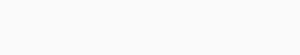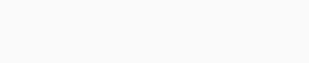# Soal dan Kunci Jawaban Sample Paper FMO 2021 grade 6

1. A store has a number of pens. If they put $$9$$ pens in a box, there is no pen left. Which answer below cannot be the number of pens in the store?

A. 2097
B. 1998
C. 3726
D. 2996
E. 945

2. A letter is called symmetrical if it can be cut into two identical parts.In the word FERMAT, how many letters are symmetrical?
A. 1
B. 3
C. 4
D. 5
E. 6

3. Ashley writes ten numbers on the black board. Ashley asks Tracy to find all prime numbers. At most how many numbers can Tracy find?A. 2
B. 3
C. 4
D. 5
E. 6

4. Lucy accidentally poured ink on her exam paper and the ink covered a number in the equation. Can you guess that number?A. 1
B. 15
C. 10
D. 8
E. 13

5. Justin has a rectangular piece of paper as follows.He folds the paper so that two red points coincide. What does the paper look like after being folded?
A.B.C.D.E.6. A group of workers had to paint a house in $$2$$ days. The first day, they worked for one third of a day. The second day, they worked for half of a day. How many hours in total did they work on painting the house?A. 20
B. 4
C. 18
D. 16
E. None of the above

7. Given a table with $$5$$ cells of different color. Which cell has the smallest value?A.  Blue
B. Yellow
C. Red
D. Green
E. White

8. Uncle Lam has a square garden. After selling $$2$$ pieces of his land, the garden looks like the figure below. Find the area of Uncle’s Lam garden right now in $$m^2$$.A. 900
B. 675
C. 750
D. 650
E. 775

9. Mr. Smith wants to go from Santa Barbara to Moonpark. He searches the train schedule online and sees the table below. How many minutes should his travel last according to this schedule?A. 105 min
B. 76 min
C. 85 min
D. 95 min
E. 75 min

10. Cindy has a rubik cube $$3\times 3\times 3$$. One day, she dropped it and broke the rubik into $$2$$ pieces. She found one piece of the rubik which looks like the figure below. At least how many small cubes does the other piece contain?A. 11
B. 16
C. 9
D. 18
E. 17

11. All students in a class took a survey about their birthday and the results were recorded in the bar graph below. Find the percentage of the number of students born in March compared to the total number of students in the class.A. 10%
B. 8%
C. 4%
D. 5%
E. None of the above

12. A rope of length 2m is glued on a cubic box so that its two ends must meet at the red point. Three sides of the box are shown below. What is the maximum surface area of the box?A. $$9600 cm^2$$
B. $$3750 cm^2$$
C. $$5400 cm^2$$
D. $$2400 cm^2$$
E. None of the above

13. Mulan fills number in a sequence of groups with pattern as below. Find the sum of all numbers in the first column of the $$25^{th}$$ group.A. 2028
B. 325
C. 2113
D. 2021
E. None of the above

14. The graph below shows Marie’s distance from home over a one-hour period, during which time she first went to the bookstore, then went to the supermarket, and then returned home. Find the average speed of Marie in the whole trip without taking the time she rests into consideration?A. 16 miles per hour
B. 12 miles per hour
C. 18 miles per hour
D. 15 miles per hour
E. None of the above

15. Nine children, numbered $$1$$ to $$9$$, sit around a circle in order. Each child has a number of balls. The child numbered $$1$$ has $$1$$ ball. The child numbered $$2$$ has $$2$$ balls. Given that the sum of the balls of any $$6$$ consecutive children is equal to $$14$$. How many balls does children numbered $$3$$ have?A. 3
B. 4
C. 5
D. 6
E. None of the above

16. A group of scientists was doing a research on a special type of water fern. They noticed that their population doubles every $$4$$ days. On $$27^{th}$$ April 2020, they started the experiment with $$3 cm^2$$ of water ferns. On $$31^{st}$$ December 2020, they distributed all the collected ferns into samples of $$10$$  $$cm^2$$ and still had $$a$$ $$cm^2$$ of water ferns left. Find the value of $$a$$.kunci jawaban